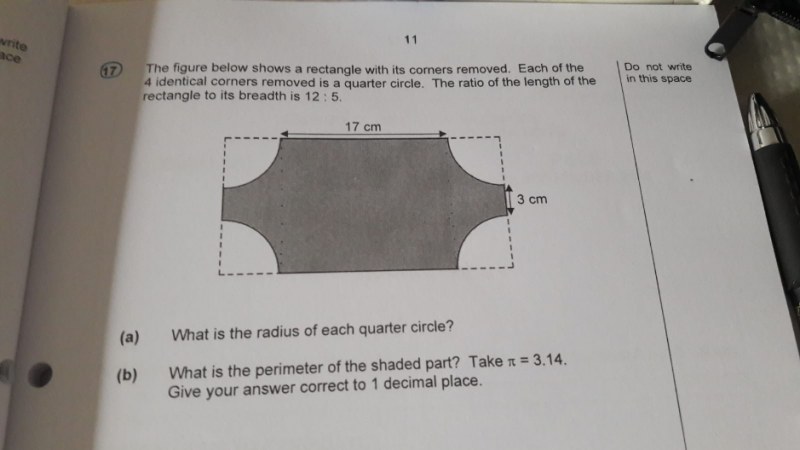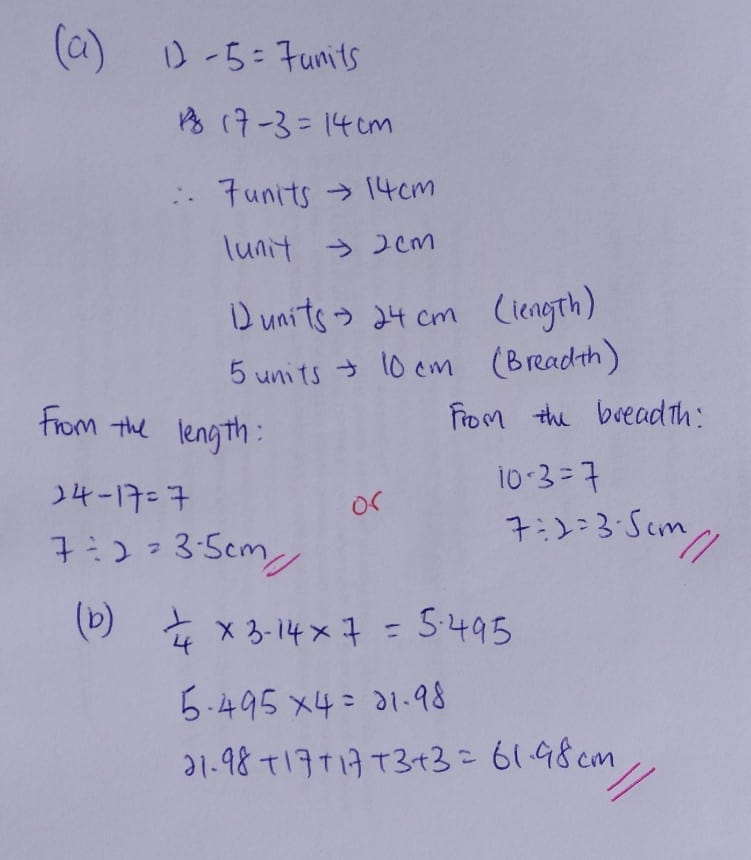# QuestionHi! I need help with this qns. Thanks!

4 Answers

# Answer

(a)
17 + 2u ——-> 12p
3 + 2u ——-> 5p
7p ——-> 14
1p ——-> 2
3 + 2u ——-> 5 x 2 = 10
1u ——-> (10 – 3)/2 = 3.5

(b)
perimeter of shaded part ——-> (3.14 x 3.5 x 2) + (17 + 3) x 2 = 62.0 (1 dp)

Ans : (a) 3.5 cm; (b) 62.0 cm (1 dp).

0 Replies 0 LikesHope this answer your question.

Owlinker
owlinker.com
FB Owlinker

0 Replies 0 Likes

(2u + 3) x 12 = (2u + 17) x 5
24u + 36 = 10u +85
24u – 10u = 85 – 36
14u = 49
1u = 49 / 14 = 3.5 cm as (a)’s answer

3.14 x 3.5 x 2 = 21.98
21.98 + 17 + 17 + 3 +3 = 61.98
= 62.0 cm as (b)’s answer

0 Replies 0 Likes